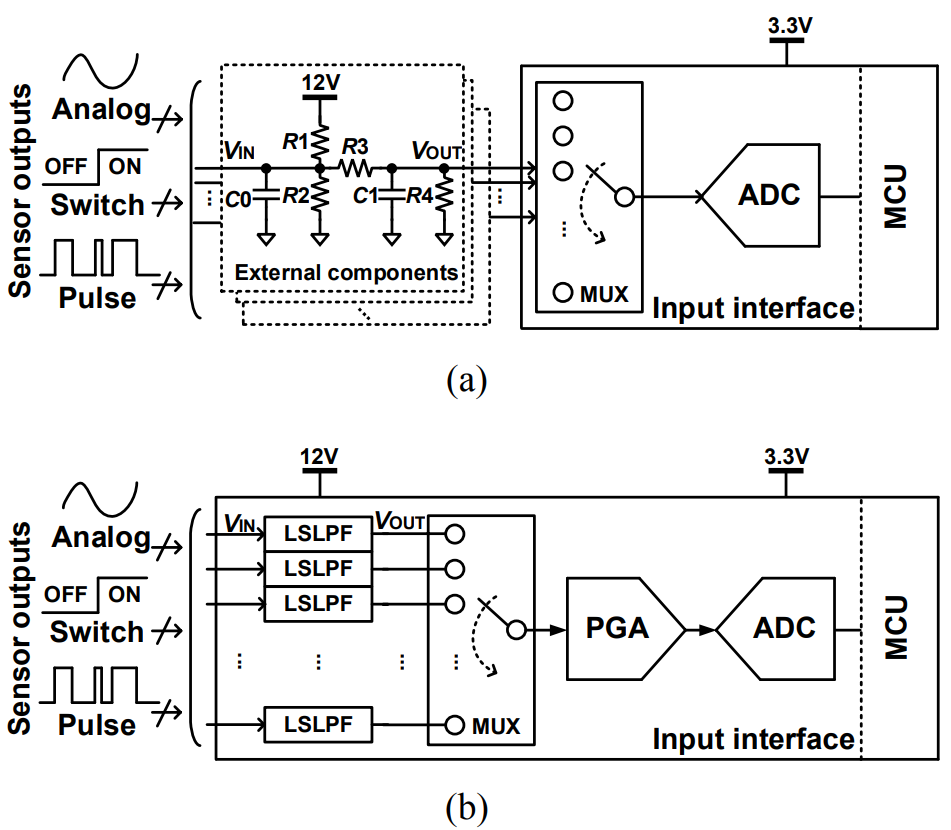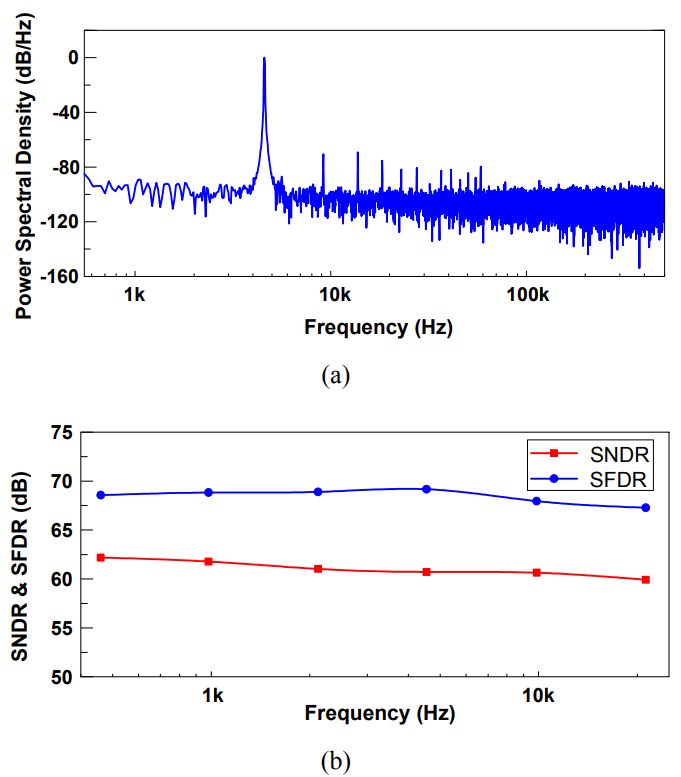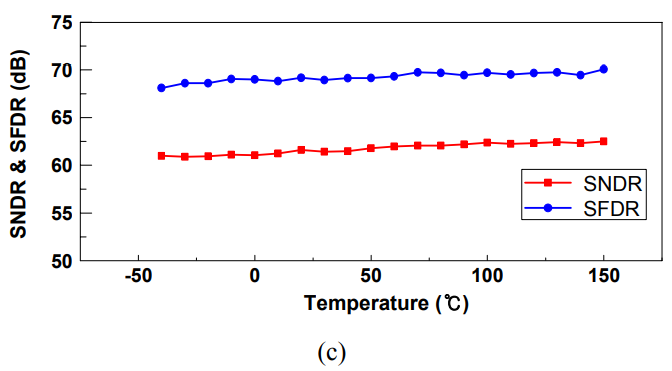Mobile QR Code1. (Department of Electrical and Computer Engineering, Seoul National University, 1, Gwanak-ro, Gwanak-gu, Seoul 08826, Korea)
2. (Gwanak Analog Co., Ltd., 1, Gwanak-ro, Gwanak-gu, Seoul 08826, Korea)

Level shifter, low-pass filter, automotive, microcontroller, input interface, analog front-end

## I. INTRODUCTION

The amount of electronics in modern motor vehicles has increased rapidly since the introduction of electronic control units (ECUs) (1). They have enabled integration which has reduced costs and increased reliability, but many challenges still remain. A typical ECU contains both digital and analog function modules including microcontrollers (MCUs), controller area network (CAN) transceivers, and input interfaces. An input interface which receives automotive sensor signals with a wide range of DC-levels consists of level-shifter, low-pass filter and an analog-front-end (AFE). The level-shifter protects the internal circuit by reducing the voltage of battery-level sensor signals, and the low-pass filter reduces the high-frequency noise from the sensor. These functions are generally merged into a single circuit with shared passive components, as shown in Fig. 1(a). Dealing with large voltage spikes and achieving a low cut-off frequency require a large capacitor, and therefore level-shifters and low-pass filters are generally implemented using external components. However, these increase the cost and reduce the robustness of the module. Sensor integrated circuit products for automotive applications are already removing external components such as capacitors for external filters (2), but previous automotive MCUs still require external components. Wang et al. (3) and Specks et al. (4) presented the MCUs with analog function modules on the same die. However, external passive components are required to protect the MCUs from battery-level voltages arriving through sensors. In addition, expensive high-quality passive elements are used for linearity. They do not provide the necessary functions such as gain control and filtering which are essential for signal processing of these signals. We present an automotive MCU constructed with 0.18 μm BCDMOS (bipolar, CMOS, and DMOS) technology with an integrated input interface that can handle analog and pulse signals as well as switches. It supports sensors with a wide range of output voltages while requiring fewer external components than previous circuits.

Fig. 1. Input interface for acquiring sensor outputs (a) conventional input interface with external components on a PCB, (b) our on-chip input interface with integrated level-shifting low-pass filter.## II. CIRCUIT IMPLEMENTATIONSitle

### 1. Input Interface

A typical input interface for automotive MCUs (5) is shown in Fig. 1(a). There is an external capacitor $C0$ to cope with surges in voltage; pull-up and pull-down resistors$R1$ and $R2$; a resistor $R3$ to limit the current reaching the MCU; a capacitor $C1$ for passive low-pass filtering of the high-frequency noise from the sensor and wiring harness; and resistors $R3$ and $R4$ form a voltage down-shifter. Fig. 1(b) is our 32-channel input interface which includes a level-shifting low-pass filter (LSLPF) and an AFE. The LSLPF reduces the amplitude of sensor signals from the battery-level voltage of 12 V to a voltage below 3.3 V and also reduces the high-frequency noise from the sensor and wiring harness.

The AFE digitizes the output of the sensor. In order to change the characteristics of each channel of the input interface to suit a particular type of sensor without requiring or trimming external components, our AFE includes various analog building-blocks. After the sensor output has been level-shifted and low-pass filtered by the LSLPF, a channel-selector with an analog multiplexer selects each channel sequentially. A PGA amplifies the output of the analog multiplexer to match the input range of a 12-bit 1 MS/s successive-approximation-register (SAR) analog-to-digital converter (ADC). In order to make full use of the full scale of the ADC, which ranges from 0.15 V to 3.15 V, the gain of the PGA can be adjusted in 5 steps of 2, 3, 4, 6, and 12 V/V.

### 2. Level-shifting Low-pass Filter

In our input interface, the function of the passive components in a conventional interface is performed by an on-chip LSLPF for each channel, as shown in Fig. 1(b). The circuit of an LSLPF is shown in Fig. 2. The diodes $D1$ and $D2$ included in the I/O pads protect the MCU from battery over-voltage. The resistors $R1$ and $R2$ form a level-shifter to reduce the peak voltage coming from a sensor from 12 V to 3 V required by the rest of the circuit. $R1$ also act as a current limiter and $R2$ acts as a pull-down resistor. $R3$ is provided when the sensor output voltage is 5 V and is brought into play when $LS_{12}$ is low. The Zener diode $D3$ is an additional safety device. When there is a simultaneous spike in $V_{\mathit{IN}}$ and the battery voltage, $D1$ is ineffective. The presence of $D3$ ensures that $V_{\mathit{OUT}}$never rises above its breakdown voltage of 5 V.

The input interface requires a low-pass filter and the low-frequency range of the output of a typical sensor requires a large capacitor. To implement this capacitor in silicon using a metal-insulator-metal (MIM) capacitor that only provides a few fF/um$^{2}$, we use a DC-decoupled capacitance-multiplication (CM) technique that utilizes the Miller effect (6,7). Our circuit consists of a single-stage amplifier made up of the transistors $MP1$ and $MN3$, a DC-decoupling capacitor $C2$, and a replica-bias generator comprising $R4$, $MP2$, and $MN7$. The charge on $C1$ is multiplied by the gain of the single-stage amplifier. $C2$ prevents DC from the sensors from reaching the amplifier while accommodating for the various output DCs of the sensor. The gate voltage of $MP1$ decoupled from the sensor output DCs is generated by the replica-bias generator. The resistor $R4$ allows the gate node of $MP1$ to offer high impedance to both the AC component of $V_{\mathit{IN}}$ and the DC component generated by $MP2$ and $MN7$.

The size of the transistor in the replica-bias generator is the same as that in the single-stage amplifier and so this scheme always operates in the saturation region regardless of the DC component of $V_{\mathit{OUT}}$. The transistors $MN1$ and $MN5$ form a source follower to increase the bandwidth of the single-stage amplifier, improving the rejection of out-of-band frequencies. The CM can be activated for each channel independently by the input signal $EN_{\mathit{CM}}$. When $LS_{12}$ and $EN_{\mathit{CM}}$ are both asserted and the gain of the source follower is approximately 1, the cut-off frequency $f_{3\mathit{dB}}$ can be derived as follows:

##### (1)
$$\frac{V_{M}}{V_{O U T}}=-g m_{M P 1}\left(r o_{M P 1} / / r o_{M N 3}\right)$$

##### (2)
\begin{aligned} C 1_{\text {Miller}} &=C 1\left(1-\frac{V_{M}}{V_{\text {OUT}}}\right) \\ &=C 1\left(1+g m_{M P 1}\left(r o_{M P 1} / / r o_{M N 3}\right)\right) \end{aligned}

##### (3)
$$f_{3 d B}=\frac{1}{2 \pi(R 1 / / R 2) C 1\left(1+g m_{M P 1}\left(r o_{M P 1} / / r o_{M N 3}\right)\right)}$$

where ‘//’ indicates that impedances are connected in parallel. $C1_{\textit{Miller}}$ represents the effective capacitance caused by Miller effect. The variable $gm_{\mathit{MP}1}$ is the transconductance of $MP1$, and $ro_{\mathit{MP}1}$ and $ro_{\mathit{MN}3}$ are the output impedances of $MP1$ and $MN3$. The gain of the amplifier is $gm_{\mathit{MP}1}\left(ro_{\mathit{MP}1}//ro_{\mathit{MN}3}\right)$.

In our design, the values of $R1$, $R2$, $R3$, and $R4$ are 79.5 kΩ, 26.5 kΩ, 92.7 kΩ, and 35 MΩ, respectively. $C1$ and $C2$ are both 2.4 pF. The gain of the single-stage amplifier is 50 dB. $V_{\mathit{B}}$ is generated by a current mirror which copies the current from an internal reference generator. The drain current through $MN3$, $MN5$, and $MN7$ is 2 μA. Therefore, the total current added in the LSLFP is only 6 μA per each channel.

The simulation result with these parameters is shown in the lower left of Fig. 2. It suggests that our CM technique can be expected to reduce $f_{3\mathit{dB}}$ from 3 MHz to 10.5 kHz. Without the CM technique, $f_{3\mathit{dB}}$ is the result of the resistors $R1$ and $R2$ and the gate capacitance of a multiplexer.

## III. MEASUREMENT RESULTS

A prototype of an automotive MCU including our LSLPFs was fabricated in 0.18 μm 1-poly 5-metal BCDMOS technology, and the input interface used 3.3 V thick-gate-oxide MOS transistors. Fig. 3 shows the die micrograph. The magnified layout shows the LSLPF which only takes up a small area. Each of the 32-channel LSLPFs occupies an area of 50 μm${\times}$320 μm which is only 0.45% of the input interface.

To assess the effectiveness of the filtering function in the LSLPF, we generated a 50 mV$_{\mathrm{pp}}$ sinusoid regarded as noise on top of 6 V DC and applied the signal to one input channel. The sinusoid passes through the LSLPF, PGA, and SAR ADC, and the output codes of the ADC can be seen as shown in Fig. 4. When the CM technology is activated, it can be seen that the 500 kHz sine wave peak-to-peak output code is reduced from about 50 LSBs to 15 LSBs, which signifies that the noise has been filtered. The Nyquist theorem indicates that the \textit{x}-axis cannot extend beyond 500 kHz. The measured signal-to-noise-plus-distortion ratio (SNDR) and the spurious-free dynamic range (SFDR) of the input interface are shown in Fig. 5(a). The SNDR remains above 60 dB up to an input frequency of 20 kHz, as shown in Fig. 5(b). In addition, the prototype was tested in the temperature range of ${-}$40 $^{\circ}$C to 150 $^{\circ}$C considering automotive applications, and the measured SNDR is maintained above 60 dB as shown in Fig. 5(c). The total current consumption of the input interface is 3.1 mA, and the current used by the LSLPF is 6 μA for each channel. Therefore, each of the 32-channel LSLPFs consumes only about 0.2% of the total current. Table 1 summarizes the key parameters of our input interface included in the automotive MCU and compares them with previous works.

## IV. CONCLUSIONS

We have shown how a LSLPF using DC-decoupled capacitance multiplication can be used in the integrated input interface of an automotive MCU to reduce the requirement for external components. Each of the 32-channel LSLPFs draws 6 μA which is only about 0.2% of the total current consumed by the input interface, and each channel takes up about 0.45% of the area of the input interface. To provide the programmability of input signals, our input interface not only receives battery-level inputs, but also provides analog-specific functions such as low-pass filtering by capacitance multiplication and gain control using a PGA. Inputs are digitized by a 12-bit 1 MS/s SAR ADC and a channel is selected using multiplexers.

Fig. 5. Measured output of the input interface (a) output spectrum for a 4.8 Vpp 4.58 kHz sinusoidal input signal, (b) SNDR and SFDR versus input frequency, (c) SNDR and SFDR versus temperature.Table 1. Key parameters of our MCU and its input interface

 Parameter This work Wang et al. (3) Specks et al. (4) Technology 0.18 μm BCDMOS 0.18 μm flash CMOS 0.18 μm HV flash CMOS Operating temperature - 40 °C to 150 °C - 40 °C to 125 °C - 40 °C to 125 °C MCU CPU 8b 8 MHz 8b 40 MHz 16b 8 MHz Memory 32 KB flash, 4 KB SRAM 128 KB flash, 6 KB SRAM, 2 KB EEPROM 60 KB flash, 2 KB SRAM, 1 KB EEPROM Input interface Supply voltage 3.3 V 1.8 V 5 V Input range 12 V 1.8 V 14 V (switch), 5 V (analog and pulse) Level-shifter 12 V or 5 V to 3 V No 14 V to 5 V (switch) Low-pass filter Internal with capacitance multiplication No No A/D conversion 32-channel 12b 1 MS/s SAR 16-channel 10b 1 MS/s SAR 12-channel 10b Analog gain Up to 12 No No Performance 60.7 dB SNDR 70.1 dB SFDR 60.64 dB SNDR 73.60 dB SFDR - Current consumption 3.1 mA - - Area Total 70.56 mm2 10.62 mm2 88 mm2 Input interface 3.51 mm2 - - 32-channel LSLPFs 0.512 mm2 - -

### ACKNOWLEDGMENTS

This work was supported by the National Research Foundation of Korea (NRF) grant funded by the Korea government (MSIT).

### REFERENCES

1
Abelein U., Lochner H., Hahn D., Straube S., Mar 2012, Complexity, quality and robustness - the challenges of tomorrow's automotive electronics, Proc. IEEE Design, automation & test in europe conference & exhibition (date), pp. 870-8712
Infineon , July 2019, TLE5045iC, TLE5046iC - XENSIV™ magnetic speed sensors – ISO 26262 compliant wheel speed sensor family, [Online]. Available: https://www.infineon.com/dgdl/Infineon-TLE5045iC_TLE5046iC-ProductBrief-v01_00-EN. pdf?fileId=5546d4626c1f3dc3016c4240743808ca3
Wang H., Deng J., Yu M., Hei Y., Nov 2012, A compact and robust MCU for automotive body applications, Proc. IEEE International Conference on Solid-State and Integrated Circuit Technology (ICSICT), pp. 1-34
Specks J. W., Broderick P., Erckert R., Harb H., Kruecken J., Feb 2000, A mixed digital-analog 16b microcontroller with 0.5Mb flash memory, on-chip power supply, physical network interface, and 40 V I/O for automotive single-chip mechatronics, in ISSCC Digest of Technical Papers, pp. 348-3495
Texas Instruments, Sep 2011, 3.3 V I/O considerations for Hercules™ safety MCUs in automotive and industrial environments, [Online]. Available: http://www.ti.com/lit/an/spna087a/ spna087a. pdf6
Rincon-Mora G. A., Jan 2000, Active capacitor multiplier in Miller-compensated circuits, IEEE Journal of Solid-State Circuits, Vol. 35, No. 1, pp. 26-327
Chen Y.C., Lu Y.C., Apr 2007, ,Capacitance multiplier circuit for PLL filter, U.S. Patent 20070090872## Author

Hyunjong Kim received the B.S. degree in the department of electrical and computer engineering from Seoul National University, Seoul, Korea, in 2014.

He is currently working towards the Ph.D. degree at the Seoul National University, Seoul, Korea.

His research interests are in analog and mixed-signal integrated circuits and systems.

Yujin Park received the B.S. and M.S. degrees in semiconductor science from Dongguk University, Seoul, Korea, in 2003 and 2005, respectively.

From 2005 to 2008, he was with Magnachip Semiconductor, Seoul, Korea.

In 2008, he joined Samsung Electronics and developed CMOS image sensor.

He received the Ph.D. degree in the department of electrical and computer engineering from the Seoul National University, Seoul, South Korea, in 2018.

His research interests are in analog and mixed-signal integrated circuits and systems.

Suhwan Kim received the B.S. and M.S. degrees in electrical engi-neering and computer science from Korea University, Seoul, Korea, in 1990 and 1992, respectively, and the Ph.D. degree in electrical engineering and computer science from the University of Michigan, Ann Arbor MI, in 2001.

From 1993 to 1999, he was with LG Electronics, Seoul Korea.

From 2001 to 2004, he was a Research Staff Member in IBM T. J. Watson Research Center, Yorktown Heights NY, USA.

In 2004, he joined Seoul National University, Seoul, South Korea, where he is currently a Professor of Electrical and Computer Engineering.

His research interests include analog and mixed-signal integrated circuits, high-speed I/O circuits, and silicon-photonic integrated circuits.

Dr. Kim has received the 1991 Best Student Paper Award of the IEEE Korea Section and the First Prize (Operational Category) in the VLSI Design Contest of the 2001 ACM/IEEE Design Automation Conference, the Best Paper Award of the 2009 Korean conference on semiconductors, and the 2011 Best Paper Award of the International Symposium on Low-Power Electronics and Design.

He served as a guest editor for the IEEE Journal of Solid-State Circuits special issue on the IEEE Asian Solid-State Circuits Conference.

He has also served as the Organizing Committee Chair for IEEE Asian Solid State Conference and General Co-chair and Technical Program Chair for the IEEE International System-on-Chip (SoC) Conference.

He has participated multiple times on the Technical Program Committee of the IEEE International SOC Conference, the International Symposium on Low-Power Electronics and Design, the IEEE Asian Solid-State Circuits Conference, and the IEEE International Solid-State Circuits Conference.

Hyunjoong Lee received the B.S., M.S., and Ph.D. degrees in electrical engineering and computer science from Seoul National University, Seoul, Korea, in 2005, 2007, and 2012, respectively.

From 2012 to 2014, he was a Postdoctoral Fellow at Seoul National University, Seoul, Korea.

From 2014 to 2016, he was a Postdoctoral Fellow at University of Toronto, Toronto, ON, Canada.

From 2016 to 2020, he was a Senior Researcher at Electronics and Tele-communications Research Institute (ETRI), Daejeon, Korea.

In 2020, he joined Gwanak Analog Co., Ltd., Seoul, Korea, where he is developing high-precision sensor interface circuits as a Principal Analog Design Engineer.

He is also a visiting associate professor at Seoul National University, Seoul, Korea.

His research interests are on CMOS analog and mixed-signal IC design, including Sigma-Delta ADC and various sensor interface circuits.